Written by Judith Buckland, RDCS, FASE, MBA

### Understanding LVH Part 2: How to Measure LV Mass and Diagnose LVH

As diagnostic imaging professionals, we often perform echocardiograms on patients with hypertension to monitor the thickness, strength and wall motion of the heart. Last week we launched our two-part blog on left ventricular hypertrophy (LVH). In part one we explained the pathophysiology behind the various categories of LVH along with the echocardiographic findings. We discussed how LVM and RWT plays a role in identifying the categories of LVH. This week we will answer the question: What is LVM and RWT and how do we obtain these values?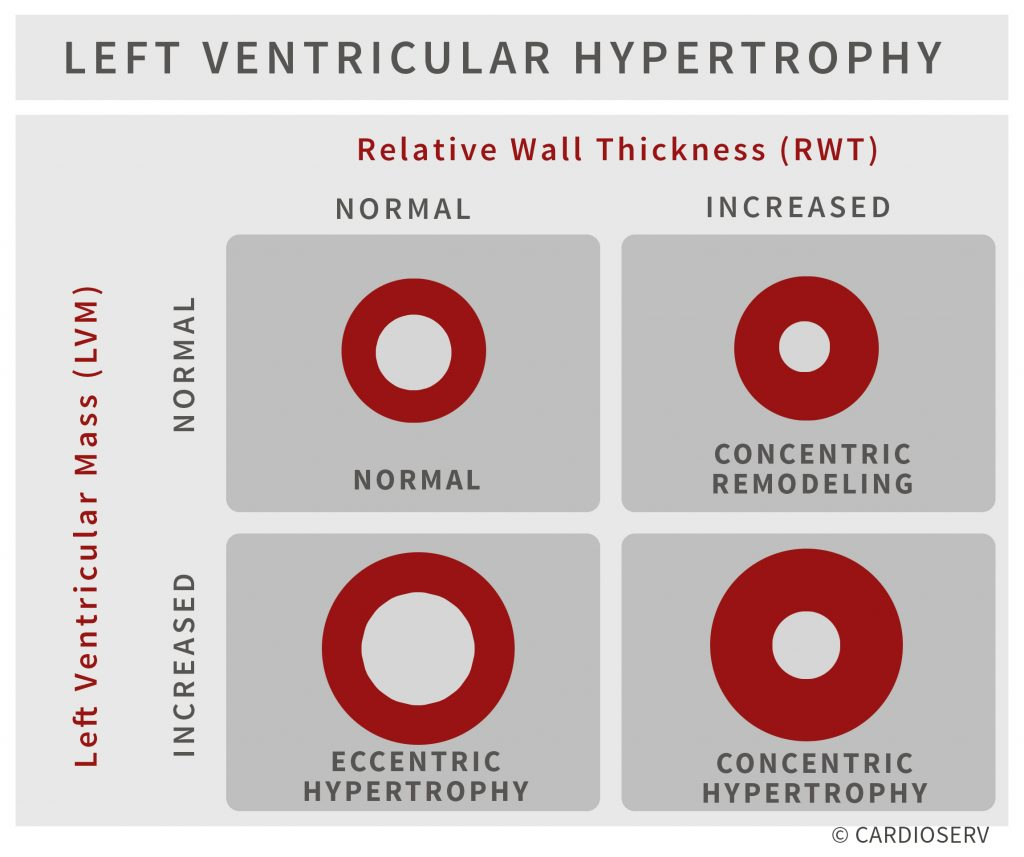# LVM and RWT

• LVM is the acronym for Left Ventricular Mass. LV mass (LVM) is a vital prognostic measurement we obtain with echocardiography to manage hypertension.
• RWT is the acronym for Relative Wall Thickness and is an additional reference value that can help further classify the type of LVH.

## How do we determine the LVM and RWT?

• Both the LVM and RWT are values calculated using a formula.
• Both formulas require accurate measurements of the LV wall thickness and LV chamber.
• There are different formulas that can be used to calculate these values based on how you measured the LV wall thickness and LV chamber size.
• We will teach the Linear ‘Cube’ Method due to:
• Ease of use
• Reproducibility
• Wealth of published data demonstrating prognostic value

# LVM: Linear ‘Cube’ Method Formula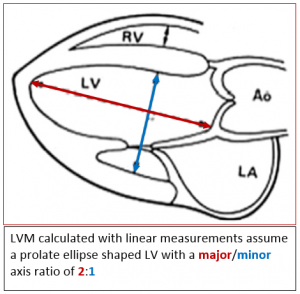All LVM algorithms are based on the subtraction of the LV cavity from the LV epicardium. The formula used for calculating the LV mass from linear dimensions assumes an ellipse shape with a major/minor axis ratio of 2:1. Needless to say, if you do not have a prolated ellipsed shaped LV this would not be the best formula to use!

Based upon the assumption of a normally shaped Left Ventricle the Cube Formula uses the following linear measurements:

1. IVS(d) – Interventricular septum diastole
2. LV(d) – Left ventricle diastole
3. PW(d) – Posterior wall diastole

ASE recommends using M-Mode in the parasternal short axis when specifically calculating the LVM but it is ok to use the linear measurements you obtain during your routine study (whether in 2D or M-Mode, PLAX or PSAX).   The advantages to M-Mode for LVM include:

• Wealth of published data
• Demonstrated prognostic value
• Simple for screening large populations

Remember that LV Mass (LVM) is a formula that your ultrasound machine will calculate for you, once you enter the measurements needed to complete the formula.  You do not need to worry about the actual formula but for all you curious folks here it is:

LVM(g) = 0.8 x 1.04 x [(IVSd + LVd + PWd)3LVd3] + 0.6g

##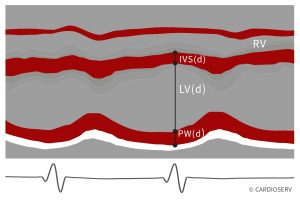• The linear Cube method should NOT be used with major distortions of the LV shape (remember the 2:1 ratio rule!)
• Preferred method for Linear Cube LVM calculations is with M-mode is the Parasternal short axis at the basal level of LV
• If using M-mode to obtain your IVS, LV, PW be sure your M-Mode is truly perpendicular to the long axis of the LV
• The formula involves cubing measurements so small mistakes are magnified
• The measurements are dependent on the image quality and the technical quality of the study

## I already measure the IVS, LV and PW but I never see the calculated LVM!

You need to make sure your ultrasound system is set up to calculate the LVM.  Usually this requires going into your configuration menu and selecting which formula you want included in your measurement package.  Remember to select the LVM Linear Cube formula.  Questions?

## LVM Reference

To quote ASE: “The main contribution of echocardiography to the management of hypertension is the assessment of left ventricular mass (LVM)”.  We need to start reporting on LV Mass on all patients with hypertension.  The normal reference range for LVM is gender specific and indexed to BSA.

• Concentric LVH = increased LVM
• Eccentric LVH = increased LVM
• Concentric remodeling = normal LVM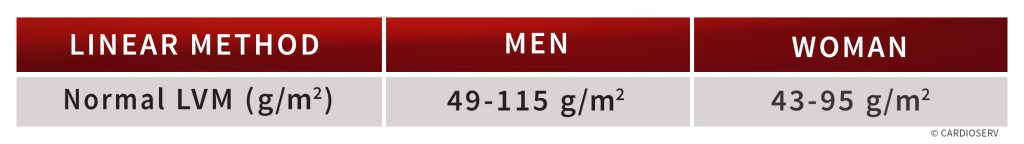# Relative Wall Thickness (RWT)

Relative Wall Thickness helps calculate if the ventricular morphology has altered.  The RWT reports the relationship between the wall thickness and cavity size.   The RWT is calculated by doubling the dimension of the posterior wall and dividing by the LV dimension.

RWT = ( 2 x PWd ) / LVd

Another variable of this equation would be to add the IVS and PW instead of multiplying the PW by two.  The reason this method is less preferred is because septal measurements may be confounded by the presence of septal bulge.

RWT = (IVSd + PWd) / LVd

Remember that Relative Wall Thickness (RWT) is also just a formula.  Your ultrasound machine will calculate this for you once you enter the measurements needed to complete the formula.  Again, you will already have obtained your posterior wall (PWd) and left ventricular dimensions (LVd).  You will just need to make sure your ultrasound system is set up to calculate and display the RWT formula.

## RWT Reference

The ASE recommends “Description of LV geometry, using at the minimum the four categories of normal geometry, concentric remodeling, and concentric and eccentric hypertrophy,
should be a standard component of the echocardiography report.”  The normal reference range for RWT is 0.32 – 0.42 regardless of gender or BSA.

• Concentric LVH = increased RWT
• Eccentric LVH = decreased RWT
• Concentric remodeling = increased RWT# Summary

• Both LV Mass (LVM) and Relative Wall Thickness (RWT) are calculations that provide you with additional information to better categorize the type of left ventricular hypertrophy.
• Both these calculations require accurate assessment of the LV cavity size and wall thickness
• This article explained the linear method (cube formula) for calculating these values
• You already measure the required elements of these formulas during a routine echo
• Adjusting the settings on your ultrasound machine will allow you to calculate these values
• Contact your ultrasound vendor (or look in the configuration menu) to display these values on your system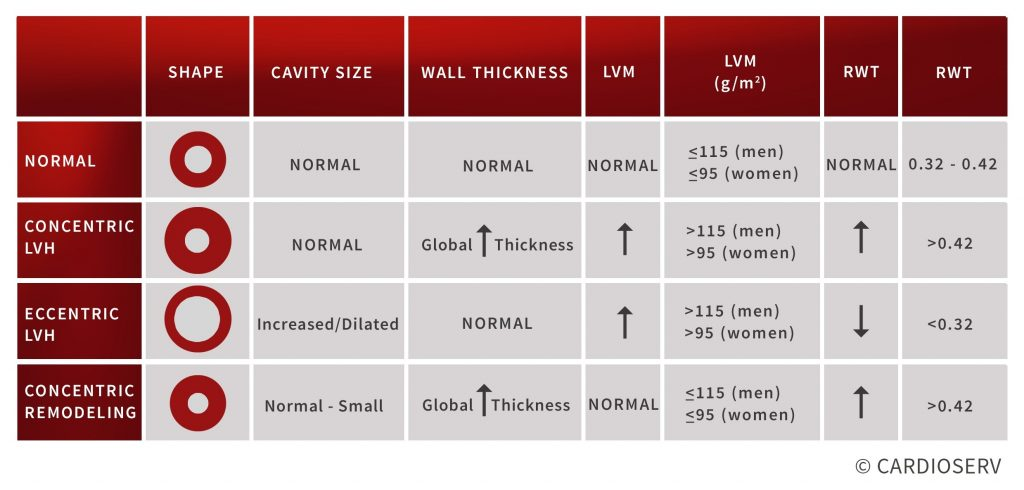# Our Services

## LET US KNOW WHAT YOU THINK...

jose donis
Very nice and simple the way to calculate the different remodel of the Hypertrophy of the left ventricle. My congratulations. Thanks a lot
Thanks for elegant discussion.
Nicolae Ciobanu
Thanks, a lot!
Emmanuel
Thank you!!!
Denise Kappes
Thank you very informative !!
Thank for bettere explaination.Is PDF of this blog available to download and read later?an you send me PDF format
Cardioserv
You're welcome! We do not have PDF downloads at this time but hope to in the future.
nidhish
this is very nice. Can you please provide the reference for NOT using cube method formula when major minor axis ratio is distorted.
Cardioserv
Thank you for your question. Please see our latest blog that discusses the 2D truncated ellipsoid method and further explains the limitation of all 2D measurements with abnormally shaped left ventricles.
Giovanni de Simone
Using body surface area to normalize LVM will misclassify obese hypertensive patients and artificially increase the proportion of concentric LV remodeling. As also recommended by EACVI/ASE (Marwick TH et al. J Am Soc Echocardiogr 2015;28:727-54.), using height raised to validated powers (called allometric signals) might be much more appropriate to identify obesity-associated LVH. The most used allometric exponent is 2.7. Normalization of LVM for height in meters raised to the power of 2.7 near doubles the popultion attributable risk of LVH.
Cardioserv
Thank you for sharing this information with our readers. We also found that article useful in explaining how height factors into LVM. The article explains how LVM and volumes bear an approximately quadratic relationship with height in men rather than approximately cubic relationship with height in women. We wrote a follow up blog to explain the relationship of height and LVM and ASE’s recommendations. Please continue to share your feedback with us and our readers. Thank you!
Lorri Guigar
Thank you for this very helpful information.
Helen
Thank you for a very helpful informarion
Lorri Guigar
Thankyou again for another great article. My question is, since the formula is to be used with the 2:1 major/minor axis, how do we calculate LVM when the heart is not 2:1,ie dilated?
gilberto garcia
very good thanks.